# Matplotlib & Seaborn入门学习

前面我们已经学习了Numpy和Pandas两个科学数据库，对这两个库已经有了初步的掌握，接下来学习的是第三个库Matplotlib和Seaborn这两个库。

Matplotlib是一个Python 2D绘图库，它以多种硬拷贝格式和跨平台的交互式环境生成出版物质量的图形。 Matplotlib可用于Python脚本，Python和IPython Shell、Jupyter笔记本，Web应用程序服务器和四个图形用户界面工具包。

首先导入相应的库。

import  matplotlib.pyplot as plt
import numpy as np

x = np.linspace(-3,3,50)
y1 = 2*x+1
y2 = x**2

# 绘制曲线
plt.figure()
plt.plot(x,y1)# num=3表示图片上方标题 变为figure3，figsize=(长，宽)设置figure大小
plt.figure(num=3,figsize=(8,5))
plt.plot(x,y2)
# 红色虚线直线宽度默认1.0
plt.plot(x,y1,color='red',linewidth=1.0,linestyle='--')
plt.show()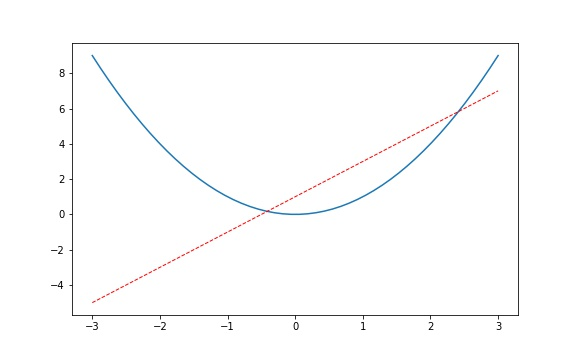## 设置坐标轴

# num=3表示图片上方标题 变为figure3，figsize=(长，宽)设置figure大小
plt.figure(num=3,figsize=(8,5))
plt.plot(x,y2)
# 红色虚线直线宽度默认1.0
plt.plot(x,y1,color='red',linewidth=1.0,linestyle='--')

plt.xlim((-1,2))#设置x轴范围
plt.ylim((-2,3))#设置轴y范围

#设置坐标轴含义， 注：英文直接写，中文需要后面加上fontproperties属性
plt.xlabel(u'价格',fontproperties='SimHei')
plt.ylabel(u'利润',fontproperties='SimHei')

# 设置x轴刻度
# -1到2区间，5个点，4个区间，平均分：[-1.,-0.25,0.5,1.25,2.]
new_ticks=np.linspace(-1,2,5)
print(new_ticks)
plt.xticks(new_ticks)

# 设置y轴刻度
'''

'''
plt.yticks([-2,-1.8,-1,1.22,3.],
['非常糟糕','糟糕',r'$good\ \alpha$',r'$really\ good$','超级好'],fontproperties='SimHei')
plt.show()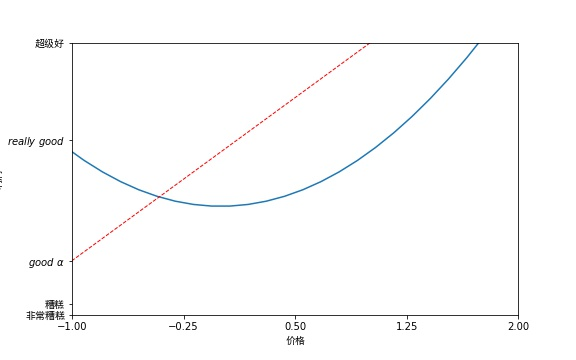# num=3表示图片上方标题 变为figure3，figsize=(长，宽)设置figure大小
plt.figure(num=3,figsize=(8,5))
plt.plot(x,y2)
# 红色虚线直线宽度默认1.0
plt.plot(x,y1,color='red',linewidth=1.0,linestyle='--')
# 设置边框/坐标轴
gca='get current axis/获取当前轴线'
ax=plt.gca()
# spines就是脊梁，即四个边框
# 取消右边与上边轴
ax.spines['right'].set_color('none')
ax.spines['top'].set_color('none')

# matlibplot并没有设置默认的x轴与y轴方向，下面就开始设置默认轴
ax.xaxis.set_ticks_position('bottom')
ax.yaxis.set_ticks_position('left')

# 设置坐标原点
# 实现将(0,-1)设为坐标原点
# 设置y轴上-1为坐标原点的y点,把x轴放置再-1处
ax.spines['bottom'].set_position(('data',-1)) # 也可以是('axes',0.1)后面是百分比，相当于定位到10%处
# 设置x轴上0为坐标原点的x点，将y轴移置0处
ax.spines['left'].set_position(('data',0))

# 再写一遍以下代码，因为以上使用set_position后，中文会显示不出来

plt.yticks([-2,-1.8,-1,1.22,3.],
['非常糟糕','糟糕',r'$good\ \alpha$',r'$really\ good$','超级好'],fontproperties='SimHei')
plt.show()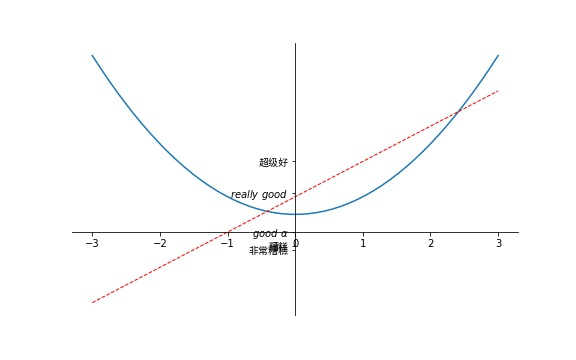## Legend图例

x=np.linspace(-3,3,50)
y1=2*x+1
y2=x**2
# 绘制直线
plt.figure()
# plt.plot(x,y1)
# 曲线与直线绘制一块
# num=3表示图片上方标题 变为figure3，figsize=(长，宽)设置figure大小
plt.figure(num=3,figsize=(8,5))

# 设置x轴范围
plt.xlim((-1,2))
# 设置轴y范围
plt.ylim((-2,3))
# 设置坐标轴含义
# 注：英文直接写，中文需要后面加上fontproperties属性
plt.xlabel(u'价格',fontproperties='SimHei')
plt.ylabel(u'利润',fontproperties='SimHei')

# -1到2区间，5个点，4个区间，平均分：[-1.,-0.25,0.5,1.25,2.]
new_ticks=np.linspace(-1,2,5)
# print(new_ticks)
plt.xticks(new_ticks)

plt.yticks([-2,-1.8,-1,1.22,3.],
['非常糟糕','糟糕',r'$good\ \alpha$',r'$really\ good$','超级好'],fontproperties='SimHei')

# 设置legend图例

l1,=plt.plot(x,y2) # 可添加label属性，只不过如果这里添加了，下面legend再添加，下面的就会覆盖此处的！
# 红色虚线直线宽度默认1.0
l2,=plt.plot(x,y1,color='red',linewidth=1.0,linestyle='--')
'''
prop={'family':'SimHei','size':15}显示中文
handles就是你给他添加legend的线,如果要用handles，则前面的plt.plot，必须用l1,形式(不要忘记逗号)

loc默认是best,给你放在一个合适的位置上，如果你拉伸弹框，位置会跟着变，自动放置合适位置
'''
plt.legend(handles=[l1,l2],prop={'family':'SimHei','size':15},loc='lower right',labels=['直线','曲线'])
plt.show()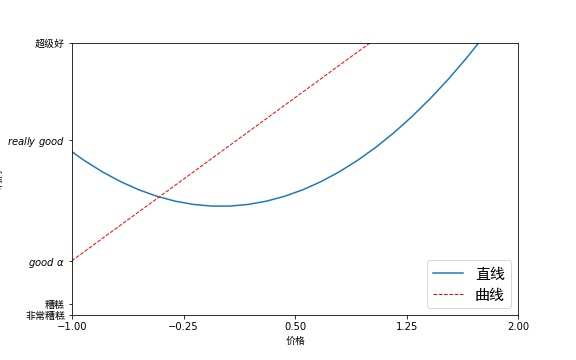## Annotation标注

import  matplotlib.pyplot as plt
import numpy as np
x=np.linspace(-3,3,20)
y=2*x+1
# 绘制直线
plt.figure(num=1,figsize=(8,5),)
plt.plot(x,y)
# gca='get current axis/获取当前轴线'
ax=plt.gca()
# spines就是脊梁，即四个边框
# 取消右边与上边轴
ax.spines['right'].set_color('none')
ax.spines['top'].set_color('none')
ax.xaxis.set_ticks_position('bottom')
ax.spines['bottom'].set_position(('data',-0)) # 也可以是('axes',0.1)后面是百分比，相当于定位到10%处
ax.yaxis.set_ticks_position('left')
ax.spines['left'].set_position(('data',0))

# 绘制特定散点
x0=1
y0=2*x0+1
# plot散点图，上述plt.plot(x,y)变为plt.scatter(x,y)绘制出来就是散点图
# s代表大小，b代表blue
plt.scatter(x0,y0,s=50,color='b')
# 把两个点放进去plot一下，画出垂直于x轴的一条线，[x0,x0]表示两个点的x,[0,y0]表示两个点的y

# 绘制(x0,y0)垂直于x轴的线

# k--表示黑色虚线，k代表黑色，--表示虚线,lw表示线宽
plt.plot([x0,x0],[0,y0],'k--',lw=2.5)
# 添加注释 annotate

'''

'''

plt.annotate(r'$2x+1=%s$'%y0,xy=(x0,y0),xycoords='data',xytext=(+30,-30),textcoords='offset points',fontsize=16,arrowprops=dict(arrowstyle='->',connectionstyle='arc3,rad=.2'))
# 添加注释 text

# 其中-3.7, 3,是选取text的位置, 空格需要用到转字符\ ,fontdict设置文本字体.
plt.text(-3.7,3,r'$This\ is\ the\ some\ text.\mu\ \sigma_i\ \alpha_t$',
fontdict={'size':'16','color':'red'})
plt.show()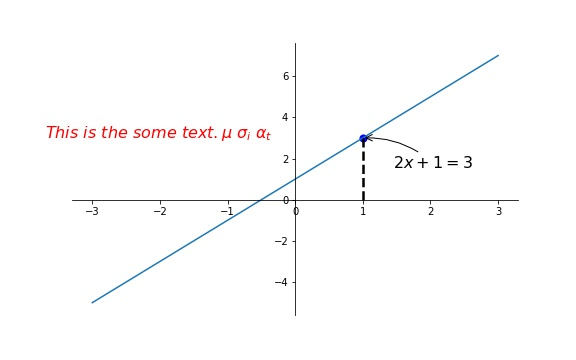## tick能见度

plt.figure()
# 设置 zorder 给 plot 在 z 轴方向排序
plt.plot(x, y, linewidth=10, zorder=1)
plt.ylim(-2, 2)
ax = plt.gca()
ax.spines['right'].set_color('none')
ax.spines['top'].set_color('none')
ax.spines['top'].set_color('none')
ax.xaxis.set_ticks_position('bottom')
ax.spines['bottom'].set_position(('data', 0))
ax.yaxis.set_ticks_position('left')
ax.spines['left'].set_position(('data', 0))

# 调整坐标

# 对被遮挡的图像调节相关透明度，本例中设置 x轴 和 y轴 的刻度数字进行透明度设置
for label in ax.get_xticklabels()+ax.get_yticklabels():
label.set_fontsize(12)
'''
其中label.set_fontsize(12)重新调节字体大小，bbox设置目的内容的透明度相关参，
facecolor调节 box 前景色，edgecolor 设置边框， 本处设置边框为无，alpha设置透明度.
'''
# 其中label.set_fontsize(12)重新调节字体大小，bbox设置目的内容的透明度相关参，
#     facecolor调节 box 前景色，edgecolor 设置边框， 本处设置边框为无，alpha设置透明度.
label.set_bbox(dict(facecolor='white',edgecolor='none',alpha=0.7))

plt.show()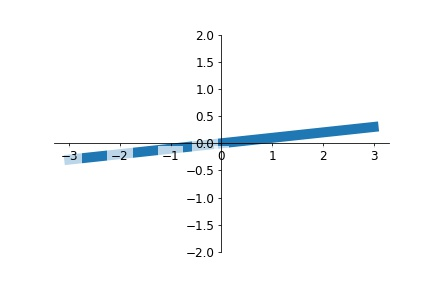## 1 Figure 和 Subplot（图和子图）

如果实在ipython里执行，可以看到一个空白的绘图窗口出现，但是在jupyter中没有任何显示，除非我们输入一些命令。plt.figure有一些选择，其中figsize保证figure有固定的大小和长宽比，这样也方便保存到磁盘中。

fig = plt.figure()
ax3 = fig.add_subplot(2, 2, 3)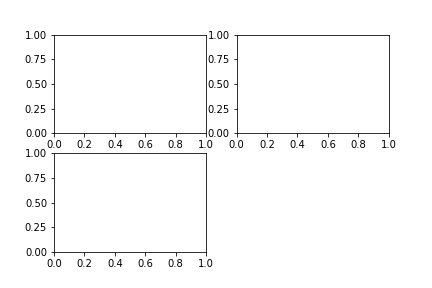plt.plot(np.random.randn(50).cumsum(), 'k--')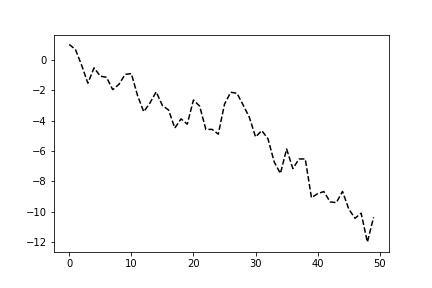_ = ax1.hist(np.random.randn(100), bins=20, color='k', alpha=0.3)
ax2.scatter(np.arange(30), np.arange(30) + 3 * np.random.randn(30))
fig

### Adjusting the spacing around subplots（调整subplot直接的间隔）

subplots_adjust(left=None, bottom=None, right=None, top=None, wspace=None, hspace=None)

wspace和hspace控制figure宽度和长度的百分比，可以用来控制subplot之间的间隔。这里有一个例子，我们让间隔为0：

fig, axes = plt.subplots(2, 2, sharex=True, sharey=True)
for i in range(2):
for j in range(2):
axes[i, j].hist(np.random.randn(500), bins=50, color='k', alpha=0.5)
plt.subplots_adjust(wspace=0, hspace=0)我们注意到轴上有些标签重叠了。matplotlib不会检查标签是否重叠，所以我们需要直接规定明确的tick location（记号位置）和tick labels（记号标签），这部分会在之后介绍。

## 2 Colors, Markers, and Line Styles（颜色，标记物，线样式）

matplotlib的plot主函数能接受x和y坐标，在可选项中，字符串能指定颜色和线样式。例如，画出x和y，用绿色的点线：

ax.plot(x, y, 'g--')

ax.plot(x, y, linestyle='--', color='g')

plt.plot(np.random.randn(30).cumsum(), 'ko--')
plt.plot(np.random.randn(30).cumsum(), color='k', linestyle='dashed', marker='o')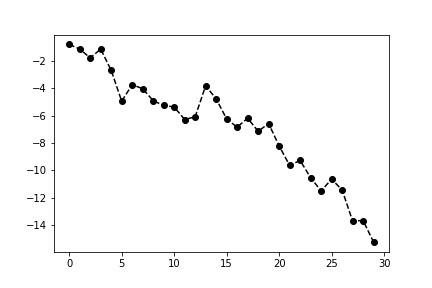data = np.random.randn(30).cumsum()
plt.plot(data, 'k--', label='Default')
plt.plot(data, 'k-', drawstyle='steps-post', label='steps-post')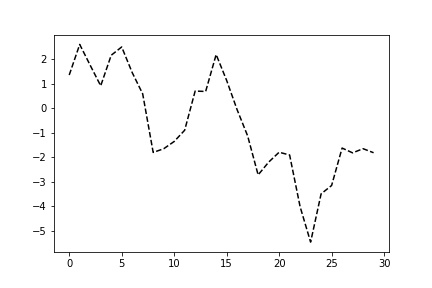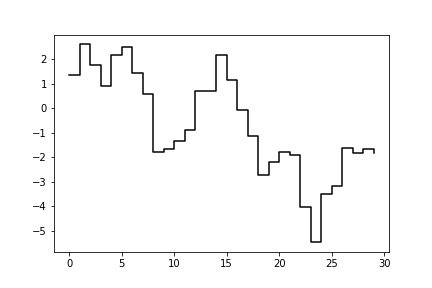当运行上面命令时，我们注意到，输出有这样的字样。这是matplotlib返回我们添加的那些子图的索引。大部分时候我们可以无视这种输出。这里，我们把label传递给了plot，这样通过plt.legend显示出每条线的意义。

注意：我们必须调用plt.legend（或ax.legend，如果有axes的话）来创建一个legend（图例），不论是非传入label。（译者：经测试，如果不调用plt.legend的话，是看不到label的）

# 3 Ticks, Labels, and Legends（标记，标签，图例）

pyplot界面是为交互式使用而设计的，它包含很多方法，比如xlim, xticks, xticklabels。这些方法控制绘图的范围，标记位置，标记标签。有两种使用方法：

• 调用的时候不传入参数，使用当前的参数设置（例如，plt.xlim()返回当前X轴的范围）
• 调用的时候传入参数，使用传入的参数设置（例如，plt.xlim([0, 10]), 令X轴的范围从0到10）

### Setting the title, axis labels, ticks, and ticklabels（设定标题，轴标签，标记，标记标签）

fig = plt.figure() # 直到下一个黑体标题出现前，不要关闭这个fig
ax.plot(np.random.randn(1000).cumsum())

为了改变x-axis tick（x轴标记），使用set_xticks和set_xticklabels。前者告诉matplotlib沿着x轴的范围，把标记放在哪里；默认会把所在位置作为标签，但我们可以用set_xticklabels来设置任意值作为标签：

ticks = ax.set_xticks([0, 250, 500, 750, 1000])
labels = ax.set_xticklabels(['one', 'two', 'three', 'four', 'five'],
rotation=30, fontsize='small')

rotation选项让x轴上的标记标签有一个30度的旋转。set_xlabel给x轴一个名字，而set_title给subplot一个标题：

ax.set_title('My first matplotlib plot')
ax.set_xlabel('Stages')

用相同的流程来更改y轴，把上面代码里的x变为y。axes类有一个set方法，能让我们一次设置很多绘图特性。对于上面的例子，我们可以写成下面这样：

props = {
'title': 'My first matplotlib plot',
'xlabel': 'Stage'
}

ax.set(**props)

from numpy.random import randn
fig = plt.figure();

ax.plot(randn(1000).cumsum(), 'k', label='one')
ax.plot(randn(1000).cumsum(), 'k--', label='two')
ax.plot(randn(1000).cumsum(), 'k.', label='three')

ax.legend(loc='best')

egend方法有一些选项，比如用loc参数设定位置。更多信息，可以参考字符串文档（ax.legend?）

loc告诉matplotlib把图例放在哪里。如果不挑剔的话，直接设定’best’就可以了，它会自动选择一个合适的位置。如果想要从图例中排除一个或更多的元素，那就不要传入label，或设置label='_nolegen_'

## 4 Annotations and Drawing on a Subplot（注释和在subplot上画图）

除了标准的绘图类型，我们可能希望画出自己的绘图注释，包括文本，箭头或其他形状。我们可以添加注释和文本，通过text，arrow，和annotate函数。text能在指定的坐标(x, y)上写出文本，还可以自己设定样式：

ax.test(x, y, 'Hello world!', family='monospace', fontsize=10)

注释可以画出文本和箭头。这里我们做一个例子，画出S&P 500指数自2007年后的价格，并用注释指出在2008~2009年经融危机期间一些重要的日期。

下面的内容可以在一个cell里直接执行：

from datetime import datetime

fig = plt.figure()

spx = data['SPX']

spx.plot(ax=ax, style='k-')

crisis_data = [
(datetime(2007, 10, 11), 'Peak of bull market'),
(datetime(2008, 3, 12), 'Bear Stearns Fails'),
(datetime(2008, 9, 15), 'Lehman Bankruptcy')
]

for date, label in crisis_data:
ax.annotate(label, xy=(date, spx.asof(date) + 75),
xytext=(date, spx.asof(date) + 225),
horizontalalignment='left', verticalalignment='top')

# Zoom in on 2007-2010
ax.set_xlim(['1/1/2007', '1/1/2011'])
ax.set_ylim([600, 1800])

ax.set_title('Important dates in the 2008-2009 financial crisis')在这幅图里，有一些点是值得强调的：ax.annotate方法能在x和y坐标指示的位置画出标签。我们可以用set_xlim和set_ylim方法来手动地设置开始和结束的边界，而不是用matplotlib的默认边界。最后，ax.set_title添加一个主标题。

可以联网看matplotlib gallery上查看更多关于标注的例子。

要想画出图形的话，更需要细心一些。matplotlib有一些对象可以用来表示一些常见的图形，被称之为patches。其中一些，比如Rectangle和Circle，在matplotlib.pyplot也有，但是全套画图形的方法还是在matplotlib.patches里。

fig = plt.figure()

rect = plt.Rectangle((0.2, 0.75), 0.4, 0.15, color='k', alpha=0.3)
circ = plt.Circle((0.7, 0.2), 0.15, color='b', alpha=0.3)
pgon = plt.Polygon([[0.15, 0.15], [0.35, 0.4], [0.2, 0.6]],
color='g', alpha=0.5)

ax.add_patch(pgon)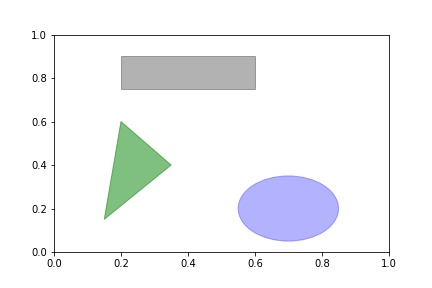## 5 Saving Plots to File（把图保存为文件）

plt.savefig('figpath.svg)

• dpi，控制每英寸长度上的分辨率
• bbox_inches, 能删除figure周围的空白部分

plt.savefig('figpath.png', dpi=400, bbox_inches='tight')

savefig不仅可以写入磁盘，还可以导出为任意像是文件一样的对象，比如BytesIO：

from io import BytesIO
buffer = BytesIO()
plt.savefig(buffer)
plot_data = buffer.getvalue()

## 6 matplotlib Configuration（matplotlib设置）

matplotlib很多默认的设置是可以自己定义的，通过修改一些全局设定，比如图大小，subplot间隔，颜色，字体大小，网格样式等等。一种容易设定的方式是用rc方法，例如，想要设置全局的图大小为10 x 10，键入：

plt.rc('figure', figsize=(10, 10))

rc中的第一个参数是我们想要自定义的组件，比如’figure’, ‘axes’, ‘xtick’, ‘ytick’, ‘grid’, ‘legend’，或其他。然后添加一个关键字来设定新的参数。一个比较方便的写法是把所有的设定写成一个dict：

font_options = {'family': 'monospace',
'weight': 'bold',
'size'  : 'small'}
plt.rc('font', **font_options)

目录